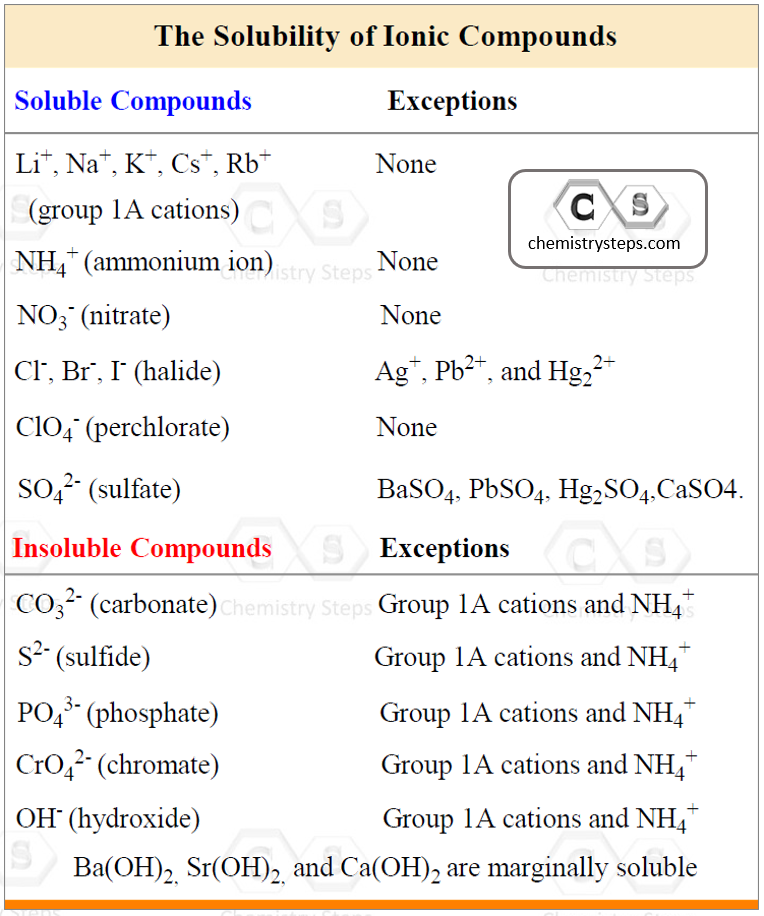## General Chemistry

All the equations where the reactants and products are shown as molecules with complete chemical formulas are called molecular equations. This, essentially, is what we use for most reactions. For example:

2K3PO4(aq) + 3BaCl2(aq) → Ba3(PO4)2(s) + 6KCl(aq)

However, we know that water-soluble ionic compounds dissociate into ions. Equations with all the soluble reactants and products dissociated into ions are called ionic equations.

When we show the electrolyte dissociated into ions, the ionic equation is obtained.

So, for the reaction above, we need to use the table for the solubility of inorganic compounds and determine which components need to be dissociated:• K3PO4 and KCl are soluble because all the salts of group 1A cations are water-soluble.
• BaCl2 is soluble because halides are soluble with the exception of their Ag+, Pb2+, and Hg22+
• Ba3(PO4)2 is insoluble because the phosphate ion falls in the category of making insoluble salts unless combined with alkali metals and ammonium ion.

Therefore, the ionic equation for this reaction is:

6K+(aq) + 2PO43-(aq) + 3Ba2+(aq) + 6Cl(aq) → Ba3(PO4)2(s) + 6K+(aq) + 6Cl(aq)

Important! Solids (precipitates), liquids, and gases do not dissociate, and we always write them in molecular forms.

Notice that some ions appear on both sides of the ionic equation. These are called spectator ions because they do not play a direct role in the reaction:

6K+(aq) + 2PO43-(aq) + 3Ba2+(aq) + 6Cl(aq) → Ba3(PO4)2(s) + 6K+(aq) + 6Cl(aq)

Spectator ions

To show the overall chemical transformation, we omit the spectator ions, and the resulting equation is called the net ionic equation:

3Ba2+(aq) + 2PO43-(aq) → Ba3(PO4)2(s)

Check Also

#### Practice

1.

Write balanced complete ionic and net ionic equations for each reaction:

a) K2SO4(aq) + CaCl2(aq) →CaSO4(s) + 2KCl(aq)

b) Na2S(aq) + 2HBr(aq) → 2NaBr(aq) + H2S(g)

c) NH4Cl(aq) + KOH(aq) → KaCl(aq) + H2O(l) + NH3(g)

d) Na2CO3(aq) + H2SO4(aq) → Na2SO4(aq) + CO2(g)+ H2O(l)

e) AgNO3(aq) + NaI(aq) → Agl(s) + NaNO3(aq)

f) HBr(aq) + KOH(aq) → KBr(aq) + H2O(l)

g) HC2H3O2(aq) + Na2CO3(aq) → NaC2H3O2(aq) + CO2(g) + H2O(l)

h) LiOH(aq) + HC2H3O2(aq) → LiC2H3O2(aq) + H2O(l)

i) HCl(aq) + NH3(aq) → NH4Cl(aq)

j) 2K3PO4(aq) + 3BaCl2(aq) → Ba3(PO4)2(s) + 6KCl(aq)

a)
This content is available to registered users only. Click here to Register! By joining Chemistry Steps, you will gain instant access to the answers and solutions for all the Practice Problems and the powerful set of General Chemistry 1 and 2 Summary Study Guides.
b)
This content is available to registered users only. Click here to Register! By joining Chemistry Steps, you will gain instant access to the answers and solutions for all the Practice Problems and the powerful set of General Chemistry 1 and 2 Summary Study Guides.
c)
This content is available to registered users only. Click here to Register! By joining Chemistry Steps, you will gain instant access to the answers and solutions for all the Practice Problems and the powerful set of General Chemistry 1 and 2 Summary Study Guides.
d)
This content is available to registered users only. Click here to Register! By joining Chemistry Steps, you will gain instant access to the answers and solutions for all the Practice Problems and the powerful set of General Chemistry 1 and 2 Summary Study Guides.
e)
This content is available to registered users only. Click here to Register! By joining Chemistry Steps, you will gain instant access to the answers and solutions for all the Practice Problems and the powerful set of General Chemistry 1 and 2 Summary Study Guides.
f)